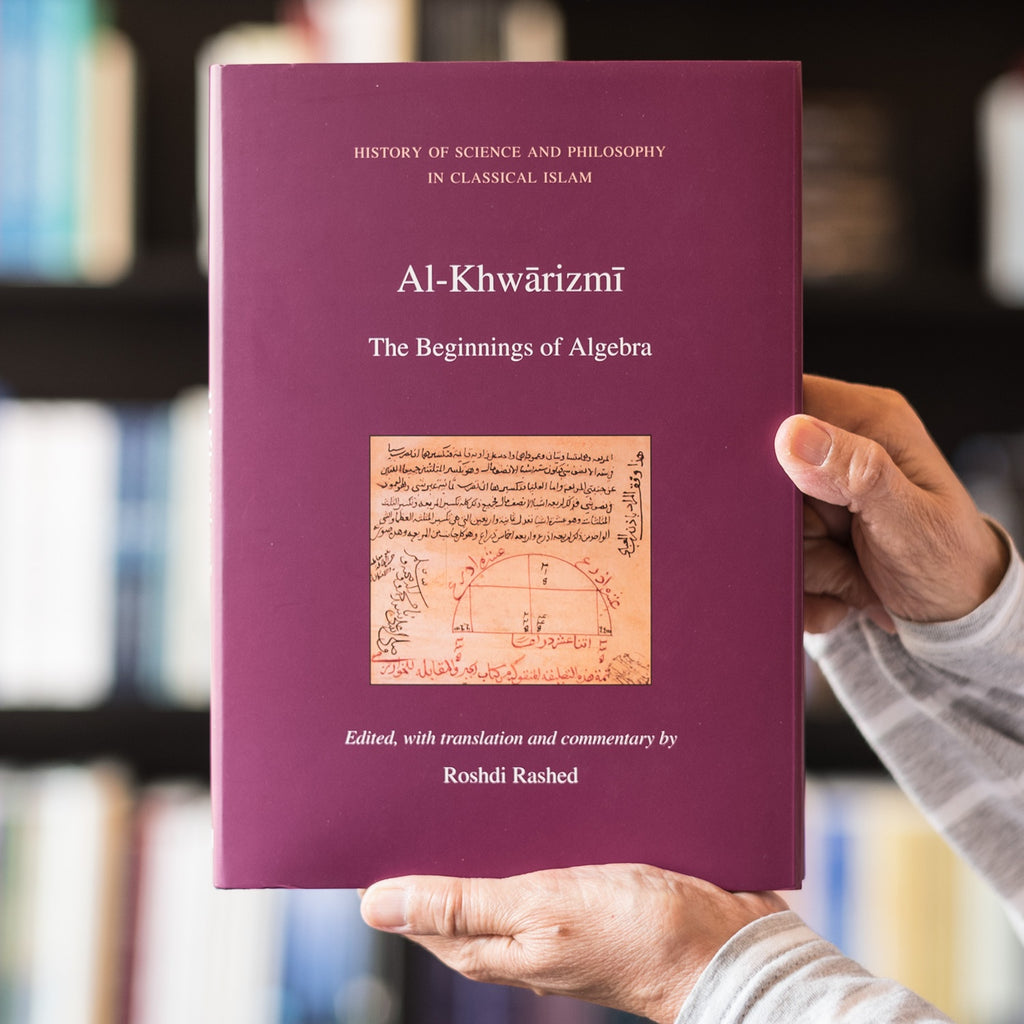Saqi

# Al-Khwarizmi: The Beginnings of Algebra

Roshdi Rashed, ed.

Hardback

9780863564307

Al-Khwarizmi was a mathematician, astronomer and geographer. He worked most of his life as a scholar in the House of Wisdom in Baghdad during the first half of the 9th century and is considered by many to be the father of algebra.

His Algebra (Kitab al-Jabr wa-al-muqabala), written around 820 AD, was the first scientific text in history to systematically present algebra as a mathematical discipline that is independent of geometry and arithmetic. This groundbreaking work is divided into two main sections: one, dealing with algebraic theory, and the other focusing on the calculation of inheritances and legacies.

Al-Khwarizmi’s book laid down the groundwork for a scientific field where mathematics and juridical learning meet, which was further developed by successive generations of mathematicians and jurists. This text also highlighted for the first time the deep-rooted possibilities in algebra to extend the use of mathematical disciplines from one to another, such as the application of arithmetic to algebra, or of geometry into algebra, and vice-versa for these three disciplines into one another; hence opening up novel areas of mathematical research.